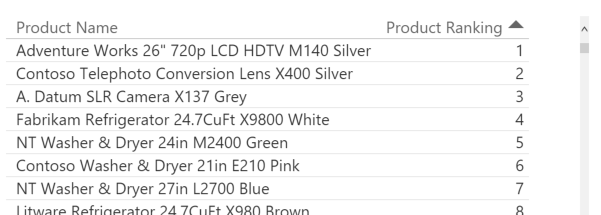The DAX language offers a function that provides the ranking of an element sorted by using a certain expression. Such a function is RANKX, it is a scalar function and it is also an iterator. The simplest use of this function is the following:

```Customer Ranking :=
RANKX ( ALL ( Customer ), [Sales Amount] )
```

The Sales measure is evaluated for every single customer, sorting them by such expression in a descendent way, and then the value of Sales computed in the filter context for Customer Ranking is compared to the values just computed for every customer, finding the position in such a sorted table. This explanation seems too long for something that is much easier to see in an example.However, this explanation is important to understand the problem that we are going to analyze. The table expression that you pass to the first argument of RANKX could be a single column table. For example, consider the following table: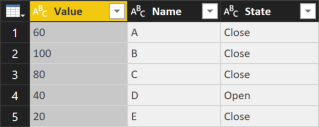The Name column has a Value numeric column that you might want to use to perform a ranking using the Sum Value measure.

```[Sum Value] :=
SUM ( Test[Value] )

[Rank Name Simple] :=
IF (
HASONEVALUE ( Test[Name] ),
RANKX ( ALL ( Test[Name] ), [Sum Value] )
)
```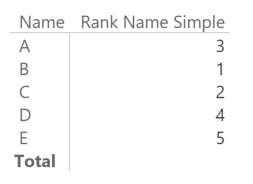If you are wondering why we should create a measure for that instead of using a calculated column, here is a simple example: you might want to display the top three names per the current filters (you might filter the State column, for example). Leveraging the fact that tables and matrixes in Power BI hides the rows that returns blank in all the displayed measures, you can obtain the desired result using such a measure:

```[Top 3 Cases] :=
IF (
[Rank Name Simple] <= 3,
[Sum Value]
)
```

You obtain this result: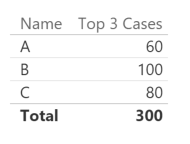However, you notice that the sort order is not what you want. In this simple case, you might simply apply the proper sort order to the column Top 3 Cases in the report, but in more complex cases the requirement could be different and the sort order should be defined by another column in the table. In this example, we want to sort the Name column by using the Value column of the same table in an ascending way. Thus, we apply the Sort By Column to the Name column, as in the following dialog box.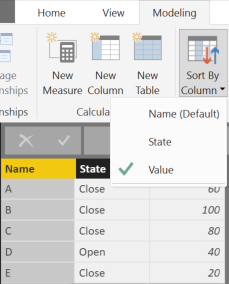At this point, our previous example no longer works, showing all the names and not just the top 3.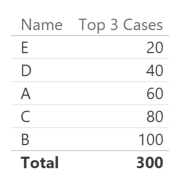In order to understand the reason of this behavior, we display the Rank Name Simple measure, too.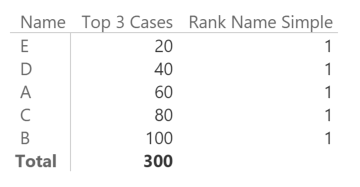The Sort By Column setting appears to break the RANKX behavior. It seems a bug, but it is not. The reason is that Power BI generates such a query for the previous report:

```EVALUATE
TOPN (
502,
SUMMARIZECOLUMNS (
ROLLUPGROUP ( 'Test'[Name], 'Test'[Value] ), "IsGrandTotalRowTotal"
),
"Top_3_Cases", 'Test'[Top 3 Cases]
),
[IsGrandTotalRowTotal], 0,
'Test'[Value], 1,
'Test'[Name], 1
)
ORDER BY
[IsGrandTotalRowTotal] DESC,
'Test'[Value],
'Test'[Name]
```

As you see, the Rank Name Simple measure is evaluated in a row context created by the iteration of two columns, Name and Value of the table Test. This happens regardless of the columns you applied to the table. In fact, we only included the Name column in the report before the measures.

Thus, when you have a Sort By Column setting active, every time you include a column in a report, Power BI also includes the corresponding sort column in the same DAX query. This is usually not an issue for any aggregation, because the two columns should share the same granularity. However, when you have an iterator over the column included in the report (Name in our example), and a context transition (the measure [Sum Value]) happening during such iterator (the RANKX function), the presence of the additional sort column (Value) in the row context that evaluates the measure generates a filter context that includes a filter over the same Value item for all the rows iterated by RANKX.

Note: for a complete understanding of the context transition, you should read the Context Transition article. A deeper explanation is also available in the book The Definitive Guide to DAX.

You can avoid this issue by including the sort column when you apply RANKX to a single column. For example, you can write:

```[Rank Name] :=
IF (
HASONEVALUE ( Test[Name] ),
RANKX ( ALL ( Test[Name], Test[Value] ), [Sum Value] )
)
```

In this way, you make sure that the context transition produced by Sum Value in RANKX will override the filter context for both Name and Value columns in the query generated by Power BI. In fact, this approach produces the expected result: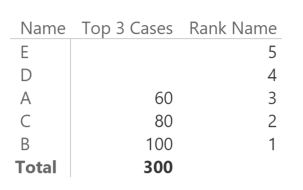Now we can see the top three names correctly if we remove from the report all the unnecessary measures.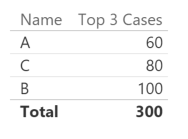Please note that Excel does not have this issue, because it relies on MDX, which applies the sort order to Name automatically without altering the semantic of the query.

Warning: the Total does not sum only the visible rows (it would be 240), but all the rows that are not visible. This is because the original Top 3 Cases measure does not check the single selection of Name. If you want to remove such a Total, you can use the following measure (I would not suggest creating a total of the visible rows because of the performance implications in a large table).

```[Top 3 Cases] :=
IF (
HASONEVALUE ( Test[Name] ),
IF (
[Rank Name Simple] <= 3,
[Sum Value]
)
)
```

If you use Power BI, keep in mind that applying a sort by column condition to the data model could potentially break an existing measure. You should know that a sort by column condition exists to generate the right measure in DAX. Iterators over physical tables should not be affected by this issue, but the table granularity could be not the right one for your calculation, and the context transition when you iterate a table can be more expensive from a performance point of view.

We could consider a best practice to set the sort by column condition in the data model before generating DAX measures using iterators over one or more columns. When you apply the sort by column setting, you should verify whether the column is used in some iterator, and whether this could affect the evaluation of the measure.